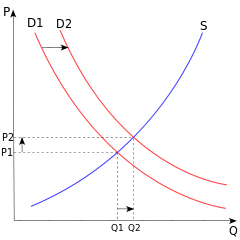# Law of demandA demand curve, shown in red and shifting to the right, demonstrating the inverse relationship between price and quantity demanded (the curve slopes downwards from left to right; higher prices reduce the quantity demanded).

In economics, the law of demand states that," all else being equal, as the price of a product increases (↑), quantity demanded falls (↓); likewise, as the price of a product decreases (↓), quantity demanded increases (↑)". In simple terms, the law of demand describes an inverse relationship, and an elasticity, between price and quantity of demand. There is a negative relationship between the quantity demanded of a good and its price. The factors held constant in this relationship are the prices of other goods and the consumer's income. There are, however, some possible exceptions to the law of demand (see Giffen goods and Veblen goods).

## Mathematical expression

Mathematically, the inverse relationship may be expressed as a causal relation:whereis the quantity demanded of good x,is the price of the good,is the demand function, andis its derivative.

Here,is the causal factor (independent variable) andIs the dependent variable.

## Graphical depiction

A demand curve is a graphical depiction that abides by the law of demand. It shows how the quantity demanded of some product during a specified period of time will change as the price of that product changes, holding all other determinants of the quantity demanded constant. Price is measured on the vertical axis and quantity demanded on the horizontal axis.

There are two important things to note about the demand curve:

• It is downward sloping indicating that between the price of a product and the quantity demanded a negative or inverse relationship exists. In other words, as the price declines the quantity demanded increases. This is indicated by a downward movement along the demand curve. An increase in price decreases the quantity demanded, and an upward movement along the demand curve occurs.
• The movement along a given demand curve due to a change in price is referred to as "change in quantity demanded". (i:e demand changes as only the price changes, and diagrammatically, there is only one demand curve,with the movement noticeable between points/see GVR notes/) the price changes, the quantity demanded changes. The term "change in demand" refers to a shift of the demand curve because of factors other than price(in the which case, prices, incomes of consumers, government policies,seasons and weather, tastes,reigning fashion, festivals etc and other non-price factors determine/cause the demand curve to shift.Diagrammatically, new curves are drawn to show the shifts, aside from the other curves/readers should note this important distinction/)

## Exceptions to the law of demand

Generally the amount demanded of a good increases with a decrease in price of the good and vice versa. In some cases, however, this may not be true. There are certain goods which do not follow this law. These include Veblen goods and Giffen goods. Further exception and details are given in the sections below.

### Giffen goods

Initially proposed by Sir Robert Giffen, economists disagree on the existence of Giffen goods in the market. A Giffen good describes an inferior good that as the price increases, demand for the product increases. As an example, during the Irish Potato Famine of the 19th century, potatoes were considered a Giffen good. Potatoes were the largest staple in the Irish diet, so as the price rose it had a large impact on income. People responded by cutting out on luxury goods such as meat and vegetables, and instead bought more potatoes. Therefore, as the price of potatoes increased, so did the quantity demanded.

### Expectation of change in the price of commodity

If an increase in the price of a commodity causes households to expect the price of a commodity to increase further, they may start purchasing a greater amount of the commodity even at the presently increased price. Similarly, if the household expects the price of the commodity to decrease, it may postpone its purchases. Thus, some argue that the law of demand is violated in such cases. In this case, the demand curve does not slope down from left to right; instead it presents a backward slope from the top right to down left. This curve is known as an exceptional demand curve.

### Basic or necessary goods

The goods which people need no matter how high the price is are basic or necessary goods. Medicines covered by insurance are a good example. An increase or decrease in the price of such a good does not affect its quantity demanded. These goods have a perfectly inelastic relationship, in that any change in price does not change the quantity demanded.

## The law of demand and change in demand

The law of demand states that, other things remaining same, the quantity demanded of a good increases when its price falls and decreases when the price rises. This is represented by movement along the demand curve, since the product's price is on the vertical axis.

The demand for goods changes as a consequence of changes in income, tastes etc. Since these factors are not on the axes of the demand curve diagram, such a change is reflected in a shift of the demand curve to a new location. A shift of the demand curve is referred to as a change in demand, in contrast to a movement along a fixed demand curve, which is referred to as a change in the quantity demanded.